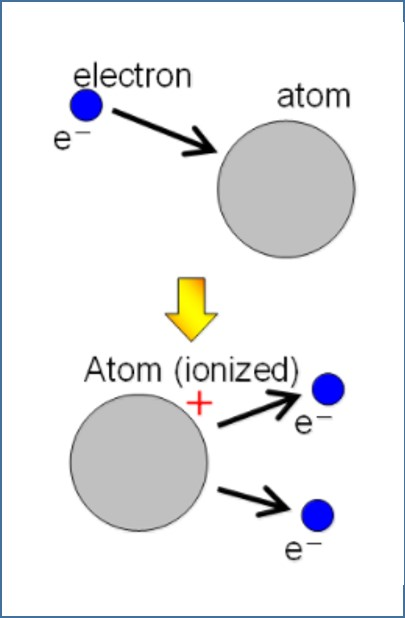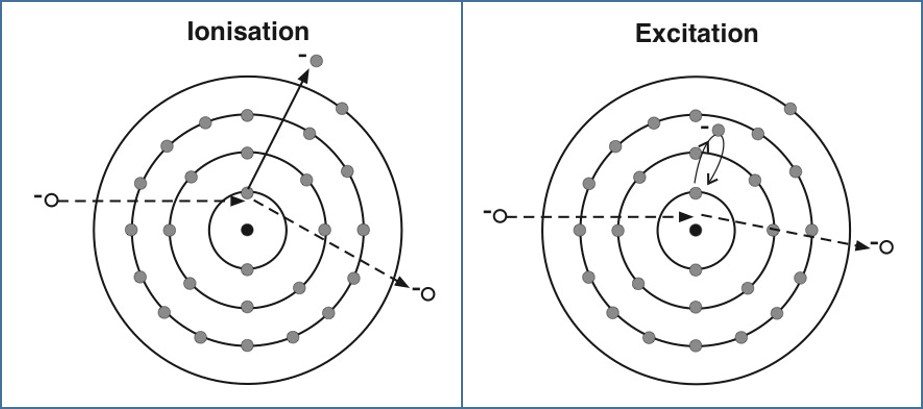# Collisions Of Electrons With Atoms

## Introduction

An electron is a subatomic particle with finite mass and negative electric charge. Electron orbits the nucleus of an atom. When an external electron collides with an atom, the energy of the moving electron gets transferred to the atom. In this article, collision of electron with atom and terms associated with the collision are explained.

A. Electron Volt

Electron volt is one of the units of energy. It is commonly used in atomic and nuclear physics. One electron volt is equal to the energy gained by an electron when the electrical potential of the electron increases by one volt. One electron volt equals 1.602 × 10−19 joule. As illustrated in Figure 1, the kinetic energy gained by an electron accelerated between the two electric poles with 1 volt potential difference is 1eV.Figure 1: Electron Volt

B. Ionisation

A normal atom is electrically neutral because it has the same number of electrons as protons. It can become a negative ion (anion) by gaining one or more electrons, or it can become a positive ion (cation) by losing one or more electrons. This process is called ionisation. This process is illustrated in the following figure.Figure 2: Ionisation

The ionisation energy (IE) is qualitatively defined as the amount of energy required to remove the most loosely bound electron of an atom. The unit of ionisation energy is the electron volt. 1 eV is the amount of energy required to remove a single electron from a single atom or molecule.

Work function, as explained in photoelectric emission, is the minimum amount of energy required to remove an electron from a solid surface.

C. Excitation

There are particular allowed orbits where electrons can exist without emitting energy. Electrons can pass between these energy levels. When electrons are given enough energy to move to higher energy levels, they get into an excited state, which is called excitation. In the ionisation process, an electron is removed from the atom. In excitation, electrons move to the higher energy levels of the same atom. The comparison between ionisation and excitation is illustrated in Figure 3.Figure 3: Comparison of Ionisation and Excitation

Excitation happens when atoms absorb energy from colliding electrons without being ionised. Excitation happens only at certain energies, according to the characteristics of the atom. If a colliding electron loses all its kinetic energy, the current due to the flow of electrons through the gas is reduced. If the colliding electron does not have enough kinetic energy to cause excitation, it is deflected by the atom, with no overall loss of kinetic energy.

D. Summary

• One electron volt is equal to the energy gained by an electron when the electrical potential at the electron increases by one volt.
• One electron volt equals 1.602 × 10−19 joules.
• In the ionisation process, an atom can become a negative ion (anion) by gaining one or more electrons, or it can become a positive ion (cation) by losing one or more electrons.
• Ionisation energy (IE) is defined as the amount of energy required to remove the most loosely bound electron of an atom.
• Work function is the minimum amount of energy required to remove an electron from a solid surface.
• When electrons are given enough energy to move to higher energy levels, they get into an excited state, which is called excitation. Excitation happens when atoms absorb energy from colliding electrons without being ionised.
• In the ionisation process, an electron is removed from the atom, whereas in the excitation process, electrons move to higher energy levels of the same atom.